## Section6.4Net Force

What happens when several forces act on a single body? For simplicity, here we consider a body of fixed mass $m \text{.}$ Second law already tells us what to do - each force will cause acceleration. The acceleration we will observe is the net acceleration. For $n$ forces, $\vec F_1\text{,}$ $\vec F_2\text{,}$ $\cdots \text{,}$ $\vec F_n\text{,}$ let the accelerations be $\vec a_1\text{,}$ $\vec a_2\text{,}$ $\cdots \text{,}$ $\vec a_n\text{,}$ respectively, the acceleration of the body will be

\begin{equation} \vec a_{\text{net}} = \vec a_1 + \vec a_2 + \cdots + \vec a_n. \label{eq-net-acceleration}\tag{6.4.1} \end{equation}

where each individual acceleration is given by the second law as

\begin{align*} \amp \vec a_1 = \dfrac{\vec F_1}{m}, \\ \amp \vec a_2 = \dfrac{\vec F_2}{m}, \\ \amp \cdots \\ \amp \vec a_n = \dfrac{\vec F_n}{m}. \end{align*}

Adding them all, we get the observed acceleration, from the sum of all forces.

\begin{equation} \vec a_{\text{net}} = \dfrac{1}{m} \left( \vec F_1 + \vec F_2 + \cdots + \vec F_n\right).\tag{6.4.2} \end{equation}

For instance, if you are pushed by two friends with equal-magnitude forces, one pointed East and the other pointed West. The net force on you will be zero and you will have no acceleration.

But, you know that if only one of your friends pushed on you, say the friend who was pushing in the East direction, you will have acceleration towards East. We say that, when both friends were pushing on you from opposite directions, each of your friend caused their own acceleration, but their vector sum cancelled out, resulting in no acceleration for you.

Now, if one friend pushed you towards East and the other towards North with an equal-magnitude force, your net acceleration will be in the North-East direction. The friend pushing East caused acceleration towards East, and the friend pushing North caused acceleration towards North.

The net result is the vector sum of the two, which is the observed acceleration. Therefore, rather than compute accelerations of each and then compute the vector sum of accelerations, we compute the vector sum of the forces, and then compute one acceleration from the net force.

We call the sum of all forces, the net force.

\begin{align} \amp \vec F_{\text{net}} = \vec F_1 + \vec F_2 + \cdots + \vec F_n,\label{eq-net-force}\tag{6.4.3} \end{align}

Often, we write $\vec a_{\text{net}}$ simply as $\vec a\text{,}$ and write the result in the following way.

\begin{equation} \vec F_{\text{net}} = m \vec a.\label{eq-net-force-equals-ma}\tag{6.4.4} \end{equation}

We may also omit $\text{net}$ from the force and indicate the net force as simply $\vec F\text{.}$

### Subsection6.4.1Free-body Diagram

If out object of interest is a point particle, all forces on that particle will act at one point, i.e., the point where the object is currently located. But, when an object has a finite size, forces on the object can act any of the outer surface and at volume points occupied by the object. The forces acting throughout the volume include gravitational, eletric, and magnetic. The forces on the surface include friction, air drag, normal force, etc.

To find the net force on a finite object, we first identify all forces at the point they act and draw them out on the body accordingly. For gravity, we assume forces acting at all volume points add up and can be placed on a special point, called center of gravity of the body. This is the first step.

But, then, to add them vectorially, especially using the analytic method of vector addition, we draw another figure in which we replace the finite body by a single point and draw force arrows coming out of that point. This new diagram is is called free-body diagram (FBD).

For instance, Figure 6.4.1 shows three forces on a block on an inclined surface - (1) weight (the name for the gravity force) $\vec W\text{,}$ is placed acting on the center of gravity, (2) normal (the upward push by the incline surface) $\vec F_N\text{,}$ and (3) friction (from the incline surface horizontal to the incline surface) $\vec F_\text{fr}\text{.}$ Corresponding free-body diagram removes the block and replaces it wth a point.

### Subsection6.4.2(Calculus) General Case

For the general case, we will need to work with the general equation, Eq. (6.2.6), in which the force would now be the net force, Eq. (6.4.3), and the momentum will the momentum of the body, $\vec p = m \vec v\text{.}$

\begin{equation*} \vec F_{\text{net}} = \dfrac{d\vec p}{dt}. \end{equation*}

We can derive this equation by setting up $n$ equations, one for each force, causing its own rate of momentum change, and then summing them all will result in net rate at which the observed momentum changes.

A box is pulled by a force of $40\text{ N}$ in the horizontal direction and a force of $30\text{ N}$ also horizontally but at an angle of $90^{\circ}$ to the direction of the other force. Find the magnitude and direction of the net force by these two forces in the horizontal plane.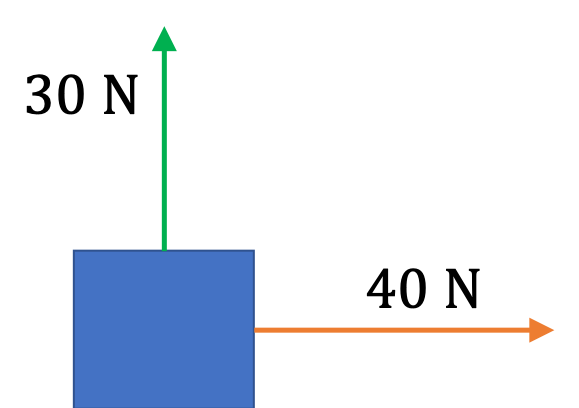Hint

Add the forces by vector rules.

$50\ N,\ 37^{\circ}$ counterclockwise from the postitive $x$ axis.

Solution

This problem can be done either graphically or analytically by setting up a coordinate system and using the components. We will do it analytically here. First, note that the forces of weight and normal force from the floor are not in the horizontal plane, therefore, we will ignore them since the question is only about forces that act in the horizontal plane.

First, we draw the forces in a figure so that the tails of the force vectors of interest are at the same point. This diagram of forces is called a free-body diagram. Then, we pick axes for a Cartesian coordinate system that would be very helpful in calculating the components as illustrated in the figure shown here.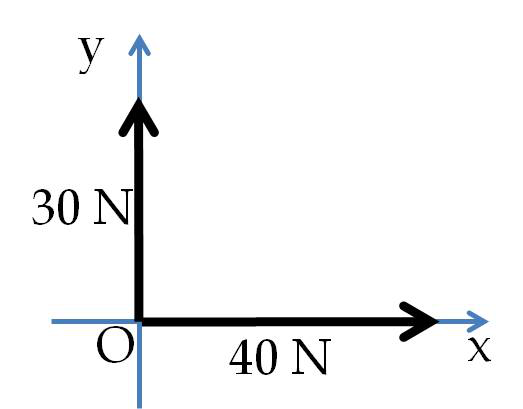Computational Steps. Using the chosen axes, we compute the Cartesian components of each force. Note that while the magnitude of a force is always positive, the components can be positive or negative. Finally, we add the $x$ components of the forces separately from their $y$ components to obtain the $x$ and $y$ components of the net force. The strength of the force is the magnitude. Therefore, we calculate the magnitude and direction of the net force from the $x$ and $y$ components as we would for any vector quantity.

The implementation in the present case. The table below summarizes the components found for each force.

 Force $x$-component $y$-component $\vec F_1$ $40\text{ N}$ $0$ $\vec F_2$ $0$ $30\text{ N}$

For simplicity in writing, let us denote the net force by $\vec F$ rather than $\vec F_{\text{net}}\text{.}$ Therefore, the $x$ and $y$ components of the net force are

\begin{align*} \amp F_x = 40 + 0 = 40\text{ N}\\ \amp F_y = 0 + 30 = 30\text{ N} \end{align*}

Therefore, the magnitude of the force is

\begin{equation*} F = \sqrt{F_x^2+F_y^2}=\sqrt{40^2+30^2} = 50\ N, \end{equation*}

and the angle from the positive $x$ axis is

\begin{equation*} \theta =\arctan\left(\frac{F_y}{F_x}\right)=\arctan\left(\frac{30}{40}\right) = 37^{\circ}. \end{equation*}

Since $(40,\ 30)$ is in the first quadrant, the direction is $37^{\circ}$ counterclockwise from the postitive $x$ axis.

The figure shows all the forces acting on a body. What is the net force on the body?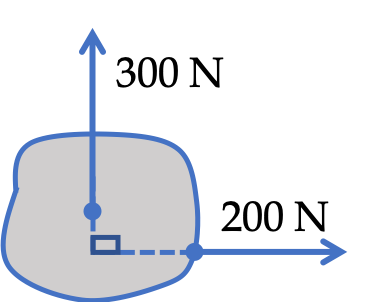Hint

You can use $x$ and $y$ axes.

$361\text{ N}$ $56.3^{\circ}$ counterclockwise from the positive $x$ axis.

Solution

We choose the positive $x$ axis to the right and the positive $y$ axis pointed up. Let us denote the horizontal force by $\vec F_1$ and the vertical force by $\vec F_2\text{.}$ Then, we have the components of the two forces as follows.

\begin{align*} \amp F_{1x} = 200\text{ N},\ \ F_{1y} = 0,\\ \amp F_{2x} = 0, \ \ F_{2y} = 300\text{ N}. \end{align*}

Let us denote the net force by $\vec F\text{.}$ Therefore, the components of the net force are

\begin{align*} \amp F_{x} = F_{1x} + F_{2x} = 200 + 0 = 200\text{ N},\\ \amp F_{x} = F_{1x} + F_{2x} = 0 + 300 = 300\text{ N}. \end{align*}

From these we get the magnitude of the net force to be

\begin{equation*} F = \sqrt{F_x^2 + F_y^2} = \sqrt{200^2 + 300^2} = 361\text{ N}. \end{equation*}

We will get the direction from the angle $\theta \text{,}$

\begin{equation*} \theta = \tan^{-1}\left(\dfrac{300}{200} \right) = 56.3^{\circ}. \end{equation*}

Since, the point $(F_x, F_y)=(200\text{ N}, 300\text{ N})$ is in the first quadrant, this angle says that, the direction of the net force is $56.3^{\circ}$ counterclockwise from the positive $x$ axis.

The figure shows all the forces acting on a body. What is the net force on the body?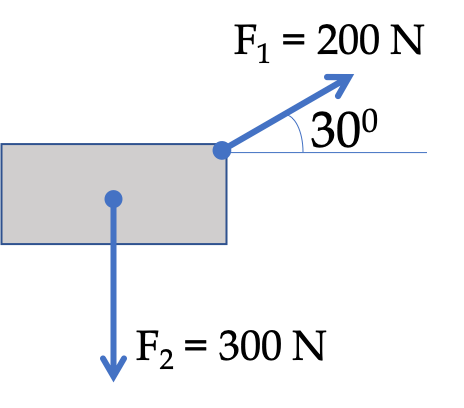Hint

You can use $x$ and $y$ axes.

$265\text{ N}$ $49.1^{\circ}$ clockwise from the positive $x$ axis.

Solution

We choose the positive $x$ axis to the right and the positive $y$ axis pointed up. Then, we have the components of the two forces as follows.

\begin{align*} \amp F_{1x} = 200\,\cos\,30^{\circ} = 173.2,\ \ F_{1y} = 200\,\sin\,30^{\circ} = 100,\\ \amp F_{2x} = 0, \ \ F_{2y} = -300\text{ N}. \end{align*}

Let us denote the net force by $\vec F\text{.}$ Therefore, the components of the net force are

\begin{align*} \amp F_{x} = F_{1x} + F_{2x} = 173.2 + 0 = 173.2\text{ N},\\ \amp F_{y} = F_{1y} + F_{2y} = 100 - 300 = -200\text{ N}. \end{align*}

From these we get the magnitude of the net force to be

\begin{equation*} F = \sqrt{F_x^2 + F_y^2} = \sqrt{173.2^2 + 200^2} = 265\text{ N}. \end{equation*}

We will get the direction from the angle $\theta \text{,}$

\begin{equation*} \theta = \tan^{-1}\left(\dfrac{-200}{173.2} \right) = -49.1^{\circ}. \end{equation*}

Since, the point $(F_x, F_y)=(173.2\text{ N}, -200\text{ N})$ is in the first quadrant, this angle says that, the direction of the net force is $49.1^{\circ}$ clockwise from the positive $x$ axis.

The figure shows all the forces acting on a body. What is the net force on the body?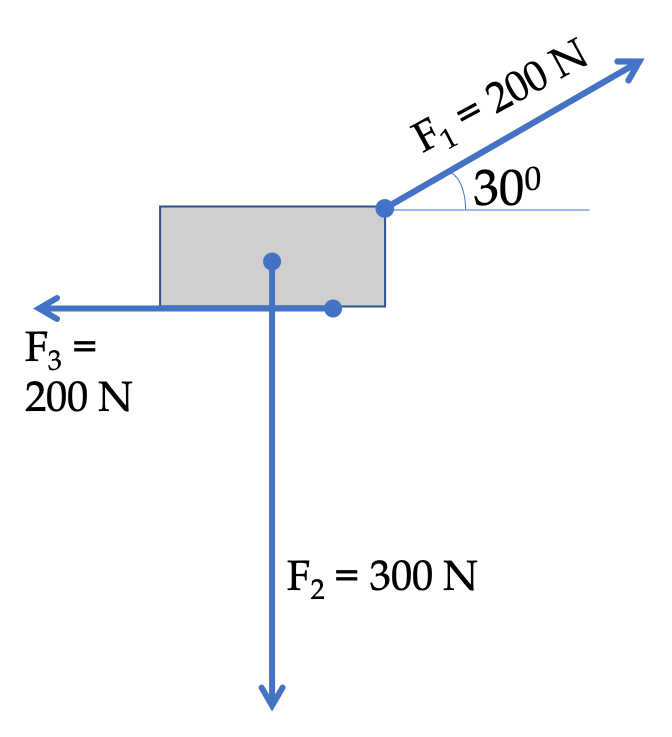Hint

You can use $x$ and $y$ axes.

$202\text{ N}$ $82.4^{\circ}$ counterclockwise from the negative $x$ axis.

Solution

We choose positive $x$ axis to the right and the positive $y$ axis pointed up. Then, we have the components of the three forces as follows.

\begin{align*} \amp F_{1x} = 200\,\cos\,30^{\circ} = 173.2,\ \ F_{1y} = 200\,\sin\,30^{\circ} = 100,\\ \amp F_{2x} = 0, \ \ F_{2y} = -300\text{ N},\\ \amp F_{3x} = -200\text{ N}, \ \ F_{2y} = 0. \end{align*}

Let us denote the net force by $\vec F\text{.}$ Therefore, the components of the net force are

\begin{align*} \amp F_{x} = F_{1x} + F_{2x} + F_{3x}= 173.2 + 0 -200= -26.8\text{ N},\\ \amp F_{x} = F_{1x} + F_{2x} + F_{3y}= 100 - 300+0 = -200\text{ N}. \end{align*}

From these we get the magnitude of the net force to be

\begin{equation*} F = \sqrt{F_x^2 + F_y^2} = \sqrt{26.8^2 + 200^2} = 202\text{ N}. \end{equation*}

We will get the direction from the angle $\theta \text{,}$

\begin{equation*} \theta = \tan^{-1}\left(\dfrac{-200}{-26.8} \right) = 82.4^{\circ}. \end{equation*}

Since, the point $(F_x, F_y)=(-26.8\text{ N}, -200\text{ N})$ is in the third quadrant, this angle says that, the direction of the net force is $82.4^{\circ}$ counterclockwise from the negative $x$ axis.

The figure shows all the forces acting on a body. The net acceleration of the body is zero. Find the magnitude and direction of the unknown force.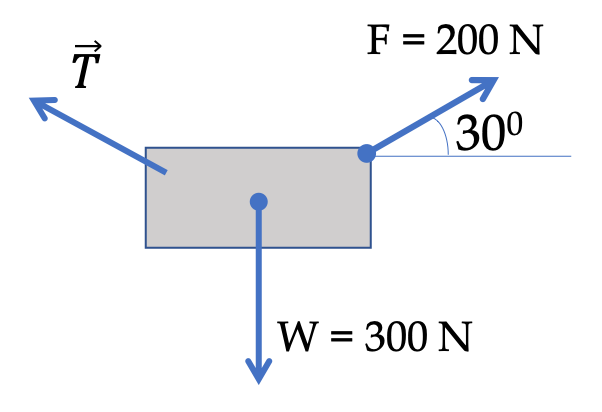Hint

Set the net force to zero.

$265\text{ N}\text{,}$ $49.1^{\circ}$ clockwise from the negative $x$ axis.

Solution

From the second law of motion, since net acceleration is zero, the net force is zero.

\begin{equation*} \vec F_{\text{net}} = 0. \end{equation*}

In a plane, this will lead to two equations along the two axes. To be concrete, we choose the positive $x$ axis to the right and the positive $y$ axis pointed up. Then, we have the components of the four forces as follows.

\begin{align*} \amp F_{x} = 200\,\cos\,30^{\circ} = 173.2,\ \ F_{y} = 200\,\sin\,30^{\circ} = 100,\\ \amp W_x = 0, \ \ W_{y} = -300.\\ \amp T_x = ?, \ \ T_{y} = ?. \end{align*}

Setting the $x$ and $y$ components of the net force to zero separately, we get the two equations.

\begin{align*} \amp 173.2 + 0 + T_x = 0,\\ \amp 100 - 300 + T_y= 0. \end{align*}

From these we get the components of $\vec T$ to be

\begin{equation*} T_x = -173.2\text{ N}, \ \ T_y = 200\text{ N} \end{equation*}

From these, we find the magnitude

\begin{equation*} T = \sqrt{T_x^2 + T_y^2} = 265\text{ N}. \end{equation*}

The angle with $x$ axis:

\begin{equation*} \theta = \tan^{-1}\left( \dfrac{200}{-173.2} \right) = -49.1^{\circ}. \end{equation*}

Since $(-173.2, 200)$ is in the second quadrant, the direction is $49.1^{\circ}$ clockwise from the negative $x$ axis.

The figure shows all the forces acting on a body. The net acceleration of the body is zero. Find the magnitudes of the two forces whose symbols are $F$ and $T \text{.}$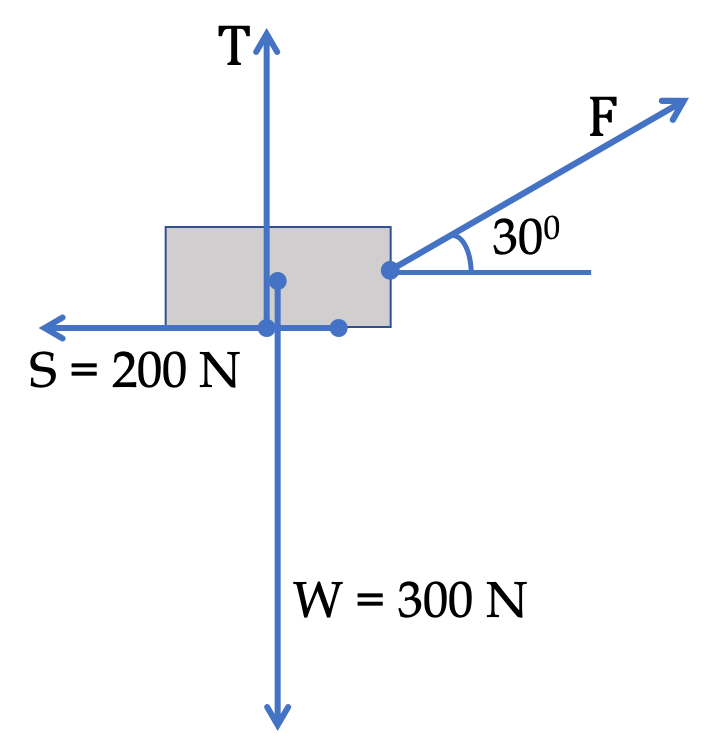Hint

Set the net force to zero.

$F = 230\text{ N}\text{,}$ $T = 194\text{ N}\text{.}$

Solution

From the second law of motion, since net acceleration is zero, the net force is zero.

\begin{equation*} \vec F_{\text{net}} = 0. \end{equation*}

In a plane, this will lead to two equations along the two axes. To be concrete, we choose the positive $x$ axis to the right and the positive $y$ axis pointed up. Then, we have the components of the four forces as follows.

\begin{align*} \amp F_{x} = F\,\cos\,30^{\circ} = 0.866\, F,\ \ F_{y} = F\,\sin\,30^{\circ} = 0.5\, F,\\ \amp T = 0, \ \ T_{y} = T,\\ \amp W_x = 0, \ \ W_{y} = -300.\\ \amp S_x = -200, \ \ S_{y} = 0. \end{align*}

Setting the $x$ and $y$ components of the net force to zero separately, we get the two equations.

\begin{align*} \amp 0.866\, F +0 +0 - 200 = 0,\\ \amp 0.5\, F + T - 300 = 0. \end{align*}

From these we get the magnitudes to be

\begin{equation*} F = 231\text{ N}, \ \ T = 194\text{ N} \end{equation*}